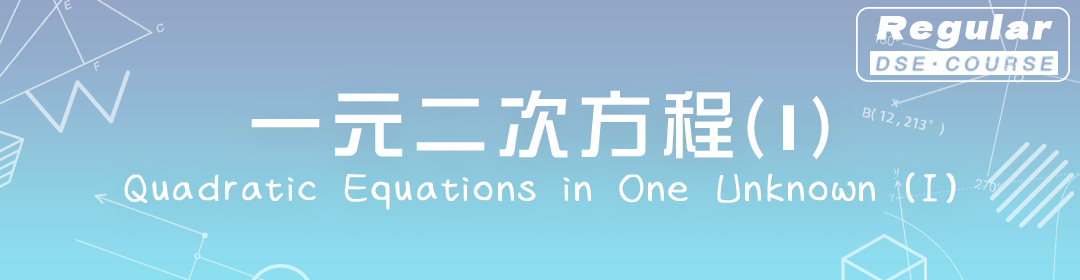DSE CH03
Quadratic Equations in one unknown (II)

- 二次方程的根的性質 Nature of Roots of a Quadratic Equations
- 二次函數的圖像 Graph of a Quadratic Function
- 由已知的根組成二次方程 Forming Quadratic Equation from Given Roots